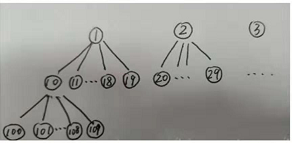## zyb的面试（广工14届）```#include <bits/stdc++.h>
using namespace std;
#define re register
#define ll long long
{
a=0;
int d=1;
char ch;
while(ch=getchar(),ch>'9'||ch<'0')
if(ch=='-')
d=-1;
a=ch-'0';
while(ch=getchar(),ch>='0'&&ch<='9')
a=a*10+ch-'0';
a*=d;
}
void write(int x)
{
if(x<0)
putchar(45),x=-x;
if(x>9)
write(x/10);
putchar(x%10+'0');
}
const int L=10;
int f[L],a[L],ten[L],cnt;///f存满十叉树的一共节点数，ten存当前层数的节点数
void geta(int n)
{
cnt=0;
while(n)
{
a[cnt++]=n%10;
n/=10;
}
}
int main()
{
f=ten=1;
for(re int i=1;i<L;i++)///初始化
{
f[i]=f[i-1]*10+1;
ten[i]=ten[i-1]*10;
}
int T;
while(T--)
{
int n,k;
geta(n);
int ans=0,cmp=0;
for(re int i=cnt-1;i>=0&&k;i--)///枚举层数
for(re int d=(ans==0);d<10;d++)///ans==0作用：因为第一位数不可能为0，所以第一个循环从1开始，之后的位数从0开始。
{
int siz=0;
if(0==cmp)
{
if(d<a[i])///若当前位置比a[i]小，说明d这颗树是有i层的满多叉树
siz=f[i];
else if(d==a[i])///相等则可能在中间某一段就停止的，就像样例中n==15，在1节点的完全多叉树中，但到15就结束了。
siz=f[i-1]+n-(ans*10+d)*ten[i]+1;///计算这颗树有多少个点
else
siz=f[i-1];
}
else if(cmp==-1)
siz=f[i];
else
siz=f[i-1];
if(k>siz)///如果k比这颗树的节点大，很明显他就在下一颗树上。
k-=siz;
else///在这一颗树上找k的位置是哪个数。
{
k--;
ans=ans*10+d;///很明显，在这颗树上的话，这颗树的第一个点肯定是d
if(0==cmp)
cmp=(d>a[i])-(d<a[i]);
break;
}
}
printf("%d\n",ans);
}
return 0;
}```
View Code

0条评论